# JP2017161685A - Image capturing lens, image capturing optical device, and digital equipment - Google Patents

Image capturing lens, image capturing optical device, and digital equipment Download PDF

## Info

Publication number
JP2017161685A
JP2017161685A JP2016045195A JP2016045195A JP2017161685A JP 2017161685 A JP2017161685 A JP 2017161685A JP 2016045195 A JP2016045195 A JP 2016045195A JP 2016045195 A JP2016045195 A JP 2016045195A JP 2017161685 A JP2017161685 A JP 2017161685A
Authority
JP
Japan
Prior art keywords
lens
lt
lens group
imaging
line
Prior art date
Legal status (The legal status is an assumption and is not a legal conclusion. Google has not performed a legal analysis and makes no representation as to the accuracy of the status listed.)
Granted
Application number
JP2016045195A
Other languages
Japanese (ja)
Other versions
JP6608738B2 (en
Inventor

Ryosuke Imajima

Yasunari Fukuda

Toshinori Take

Takayuki Sensui

Original Assignee
コニカミノルタ株式会社
Konica Minolta Inc

Nikon Corp
Priority date (The priority date is an assumption and is not a legal conclusion. Google has not performed a legal analysis and makes no representation as to the accuracy of the date listed.)
Filing date
Publication date
Application filed by コニカミノルタ株式会社, Konica Minolta Inc, 株式会社ニコン, Nikon Corp filed Critical コニカミノルタ株式会社
Priority to JP2016045195A priority Critical patent/JP6608738B2/en
Priority claimed from JP2016045195A external-priority patent/JP6608738B2/en
Publication of JP2017161685A publication Critical patent/JP2017161685A/en
Application granted granted Critical
Publication of JP6608738B2 publication Critical patent/JP6608738B2/en
Application status is Active legal-status Critical
Anticipated expiration legal-status Critical

• 230000003287 optical Effects 0 abstract title 3
• 230000004075 alteration Effects 0 abstract 1
• 230000014509 gene expression Effects 0 abstract 1
• 230000001629 suppression Effects 0 abstract 1

## Images

•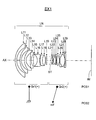•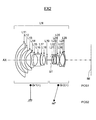•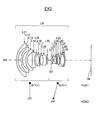••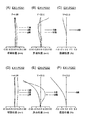•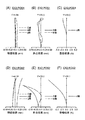•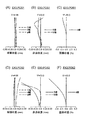•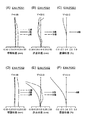•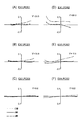••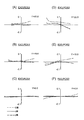•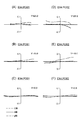•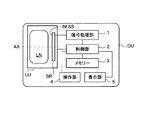## Abstract

An imaging lens in which fluctuations in optical performance due to focusing are suppressed and various aberrations are satisfactorily corrected up to a high angle of view, and an imaging optical apparatus including the same are an ultra-wide angle lens having a large ratio of back focus to focal length And provide digital equipment. An imaging lens LN includes, in order from the object side, a first lens group Gr1 having a positive power and a second lens group Gr2 having a positive power. The first lens group Gr1 is negative in order from the object side. It consists of first to fourth lenses L1 to L4 having negative and negative powers. During focusing on a short-distance object, the first lens group Gr1 is fixed with respect to the image plane IM, the second lens group Gr2 moves toward the object side, and the conditional expression: 0.2 <Φ1 / Φ <0. 5, 0.2 <Φ2 / Φ <0.3, 0.6 <Φ1 / Φ2 <1.7 (Φ1: Power of the first lens group, Φ2: Power of the second lens group, Φ: Power of the entire system ) Is satisfied. [Selection] Figure 1

## Description

The present invention relates to an imaging lens, an imaging optical device, and a digital device. For example, an image of a subject is captured by an imaging device (for example, a CCD (Charge Coupled Device) type image sensor, a CMOS (Complementary Metal-Oxide Semiconductor) type image sensor, or the like. A compact and ultra-wide-angle imaging lens suitable for an interchangeable lens digital camera captured by a solid-state imaging device), an imaging optical device that outputs an image of a subject captured by the imaging lens and the imaging device as an electrical signal, and The present invention relates to a digital device with an image input function such as a digital camera equipped with an imaging optical device.

Patent Documents 1 and 2 propose an ultra wide-angle imaging lens as an interchangeable lens for an interchangeable lens digital camera. Such a wide-angle lens requires a relatively long back focus. Further, when the lens shift mechanism is disposed on the lens back side, it is necessary to ensure a longer back focus than an imaging lens for a normal single-lens reflex camera. Moreover, in order to cope with the displacement of the image plane due to the lens shift, it is necessary to enlarge the image circle, so that the required angle of view is further increased.

As a wide-angle lens in which the ratio of the back focus to the focal length is large, a retrofocus type wide-angle lens preceded by a negative lens group is generally known. In a retrofocus type wide-angle lens, since a negative lens group and a positive lens group are arranged in order from the object side and become an asymmetric type as a whole, the amount of various aberrations increases, making it difficult to correct aberrations. In addition, since aberration fluctuation due to focus also increases, it becomes difficult to achieve high optical performance over the entire object distance. For this reason, the floating of the focus group is generally used as a technique for maintaining high imaging performance from infinity to a close distance while suppressing fluctuations in the image plane during focusing.

JP 2010-191077 A JP 2011-102863 A

The wide-angle lens described in Patent Document 1 maintains high imaging performance from infinity to a close distance, but the configuration is complicated to perform floating. The wide-angle lens described in Patent Document 2 has high imaging performance up to a high angle of view. However, if the back focus is further increased, it is difficult to maintain high imaging performance up to a high angle of view. Become.

The present invention has been made in view of such a situation, and the object thereof is an ultra-wide-angle lens having a large ratio of a back focus to a focal length. An object of the present invention is to provide an imaging lens in which various aberrations are well corrected, an imaging optical device including the imaging lens, and a digital device.

In order to achieve the above object, the imaging lens of the first invention comprises, in order from the object side, a first lens group having a positive power and a second lens group having a positive power,
The first lens group, in order from the object side, has at least a first lens with negative power, a second lens with negative power, a third lens with negative power, and a fourth lens with negative power,
When focusing on a short-distance object, the first lens group is fixed with respect to the image plane, and the second lens group moves toward the object side,
The following conditional expressions (1) to (3) are satisfied.
0.2 <Φ1 / Φ <0.5 (1)
0.2 <Φ2 / Φ <0.3 (2)
0.6 <Φ1 / Φ2 <1.7 (3)
However,
Φ1: Power of the first lens group,
Φ2: Power of the second lens group,
Φ: Power of the whole system,
It is.

The imaging lens according to a second aspect of the present invention is the imaging lens according to the first aspect, wherein at least one of the first to fourth lenses has an aspherical shape with a negative power that decreases from the center of the lens toward the lens outer periphery. A negative meniscus lens.

The imaging lens of a third invention is characterized in that, in the first or second invention, the lens located closest to the image side in the second lens group has an aspherical surface on at least one surface.

The imaging lens of 4th invention contains the cemented lens which satisfies the following conditional expressions (4)-(6) in said 1st lens group in any one said 1st-3rd invention. Features.
-1.5 <R1a / f <0 (4)
-0.3 <nL1a1-nL1a2 <-0.2 (5)
0 <νL1a1-νL1a2 <10 (6)
However,
R1a: radius of curvature of the joint surface,
f: focal length of the entire system,
nL1a1: a refractive index with respect to d-line of a positive lens having a cemented surface that satisfies the conditional expression (4),
nL1a2: a refractive index related to the d-line of a negative lens having a cemented surface that satisfies the conditional expression (4),
νL1a1: Abbe number related to the d-line of a positive lens having a cemented surface that satisfies conditional expression (4),
νL1a2: Abbe number related to the d-line of a negative lens having a cemented surface satisfying conditional expression (4),
It is.

The imaging lens of 5th invention contains the cemented lens which satisfies the following conditional expressions (7)-(9) in said 1st lens group in any one of said 1st-4th invention. Features.
0 <R1b / f <1.5 (7)
-0.25 <nL1b1-nL1b2 <-0.1 (8)
−10 <νL1b1-νL1b2 <12 (9)
However,
R1b: radius of curvature of the joint surface,
f: focal length of the entire system,
nL1b1: Refractive index related to d-line of a positive lens having a cemented surface that satisfies conditional expression (7),
nL1b2: a refractive index related to the d-line of a negative lens having a cemented surface that satisfies the conditional expression (7),
νL1b1: Abbe number related to the d-line of a positive lens having a cemented surface that satisfies conditional expression (7),
νL1b2: Abbe number related to the d-line of a negative lens having a cemented surface that satisfies conditional expression (7),
It is.

An imaging lens according to a sixth aspect of the present invention is the imaging lens according to any one of the first to fifth aspects, wherein the first lens group includes two or more cemented lenses closer to the image plane than the first to fourth lenses. As a lens that includes the following conditional expressions (10) and (11) and at least one negative lens that satisfies the following conditional expressions (10) and (11): Features.
(0.6425- [theta] gf) / [nu] d> 0.00150 (10)
νd <40 (11)
However,
θgf: partial dispersion ratio of the lens material,
θgf = (Ng−NF) / (NF−NC)
Ng: refractive index for g-line,
NF: refractive index for F-line,
NC: Refractive index for C-line,
νd: Abbe number related to the d-line of the lens material,
It is.

An imaging lens of a seventh invention is the imaging lens of any one of the first to sixth inventions, wherein the second lens group has at least three positive lenses satisfying the following conditional expressions (12) and (13): It is characterized by that.
(0.6425- [theta] gf) / [nu] d <0.00150 (12)
νd> 30 (13)
However,
θgf: partial dispersion ratio of the lens material,
θgf = (Ng−NF) / (NF−NC)
Ng: refractive index for g-line,
NF: refractive index for F-line,
NC: Refractive index for C-line,
νd: Abbe number related to the d-line of the lens material,
It is.

An imaging optical device according to an eighth invention includes the imaging lens according to any one of the first to seventh inventions, and an imaging element that converts an optical image formed on the imaging surface into an electrical signal. And the imaging lens is provided so that an optical image of a subject is formed on the imaging surface of the imaging device.

According to a ninth aspect of the present invention, there is provided a digital apparatus including the imaging optical device according to the eighth aspect, to which at least one function of still image shooting and moving image shooting of a subject is added.

According to the present invention, although the ratio of the back focus BF to the focal length f is large (BF / f ≧ 2), the optical performance fluctuation due to focusing can be suppressed and various aberrations can be corrected well up to a high angle of view. An image pickup lens and an image pickup optical device that have been provided can be realized. By using the imaging lens or the imaging optical device for a digital device (for example, a digital camera), a high-performance image input function can be added to the digital device in a compact manner.

The lens block diagram of 1st Embodiment (Example 1). The lens block diagram of 2nd Embodiment (Example 2). The lens block diagram of 3rd Embodiment (Example 3). The lens block diagram of 4th Embodiment (Example 4). FIG. 3 is a longitudinal aberration diagram of Example 1. FIG. 6 is a longitudinal aberration diagram of Example 2. FIG. 6 is a longitudinal aberration diagram of Example 3. FIG. 6 is a longitudinal aberration diagram of Example 4. FIG. 4 is a lateral aberration diagram of Example 1. FIG. 4 is a lateral aberration diagram of Example 2. FIG. 4 is a lateral aberration diagram of Example 3. FIG. 6 is a lateral aberration diagram of Example 4. FIG. 3 is a schematic diagram illustrating a schematic configuration example of a digital device equipped with an imaging optical device.

Hereinafter, an imaging lens, an imaging optical device, and a digital device according to embodiments of the present invention will be described. The imaging lens according to the embodiment of the present invention includes, in order from the object side, a first lens group having a positive power and a second lens group having a positive power (power: an amount defined by the reciprocal of the focal length). The first lens group includes at least a first lens having a negative power, a second lens having a negative power, a third lens having a negative power, and a fourth lens having a negative power, in order from the object side. When focusing on a short-distance object, the first lens group is fixed with respect to the image plane, and the second lens group moves to the object side. And it is characterized by satisfying the following conditional expressions (1) to (3).
0.2 <Φ1 / Φ <0.5 (1)
0.2 <Φ2 / Φ <0.3 (2)
0.6 <Φ1 / Φ2 <1.7 (3)
However,
Φ1: Power of the first lens group,
Φ2: Power of the second lens group,
Φ: Power of the whole system,
It is.

In a retrofocus type wide-angle lens in which the ratio BF / f of the back focus BF to the focal length f of the entire system is large, in order to increase the ratio, the negative power of the preceding negative lens group is increased to increase the axial ratio. It is necessary to increase the diameter of the light beam. In addition, a lens group with a strong positive power is placed after the negative lens group in order to collect the light beam spread with a strong negative power and correct the large negative distortion generated in the negative lens group. There is a need. The first lens group includes four lenses having negative, negative and negative powers in order from the object side, and the four lenses are used as a negative lens group to constitute a retrofocus type having a negative and positive power arrangement. doing.

Conditional expression (1) defines a preferable condition range regarding the power of the first lens group from the viewpoint of retrofocus type power arrangement. If the upper limit of conditional expression (1) is exceeded, the negative power of the preceding negative lens group in the first lens group becomes weak, and the ratio BF / f of the back focus with respect to the focal length becomes small. If the lower limit of conditional expression (1) is not reached, the negative power of the preceding negative lens group in the first lens group becomes strong, and it becomes difficult to correct negative distortion and the like generated in the negative lens group. Further, since it is necessary to increase the positive power of the positive lens group to be subsequently arranged, the overall asymmetry is increased, and it becomes difficult to correct coma aberration, curvature of field, and the like. Therefore, by satisfying conditional expression (1), it is possible to achieve a good balance between securing a long back focus and improving aberration performance.

Conditional expression (2) defines a preferable condition range regarding the power of the second lens group from the viewpoint of retrofocus type power arrangement. If the upper limit of conditional expression (2) is exceeded, the power of the positive lens in the second lens group becomes strong, but if a lens made of a high refractive index material is used as the positive lens, it becomes difficult to correct chromatic aberration. When using a lens with a positive anomalous partially dispersive material that can correct chromatic aberration well, the curvature of the lens surface must be increased to obtain the desired power, resulting in spherical aberration and field curvature. Is likely to occur. If the lower limit of conditional expression (2) is not reached, the amount of movement at the time of focusing increases, and when the second lens group moves for focusing, the height of the off-axis ray passing through the second lens group varies greatly. It will be. As a result, it becomes difficult to suppress fluctuations in field curvature and coma due to focusing. Therefore, by satisfying conditional expression (2), it becomes possible to satisfactorily correct various aberrations up to a high angle of view by suppressing fluctuations in optical performance due to focusing while securing a long back focus.

Conditional expression (3) defines a preferable range of the power ratio between the first lens group and the second lens group from the viewpoint of retrofocus type power arrangement. If the upper limit of conditional expression (3) is exceeded, the negative power of the preceding negative lens group in the first lens group becomes weak, and the ratio BF / f of the back focus with respect to the focal length becomes small. Further, the amount of movement during focusing increases due to the weak power of the second lens group, and when the second lens group moves for focusing, the height of the off-axis ray passing through the second lens group is large. Will fluctuate. As a result, it becomes difficult to suppress fluctuations in field curvature and coma due to focusing.

If the lower limit of conditional expression (3) is not reached, the negative power of the preceding negative lens group in the first lens group becomes strong, and it becomes difficult to correct negative distortion and the like generated in the negative lens group. Further, since it is necessary to increase the positive power of the positive lens group to be subsequently arranged, the overall asymmetry is increased, and it becomes difficult to correct coma aberration, curvature of field, and the like. Further, when the power of the positive lens in the second lens group becomes strong, if a lens made of a high refractive index material is used as the positive lens, it becomes difficult to correct chromatic aberration. When using a lens with a positive anomalous partially dispersive material that can correct chromatic aberration well, the curvature of the lens surface must be increased to obtain the desired power, resulting in spherical aberration and field curvature. Is likely to occur.

Therefore, by ensuring that the lenses in the first lens group have at least negative, negative and negative power arrangements in order from the object side and satisfy the conditional expressions (1) to (3), long back focus is ensured and aberration performance is improved. Improvement can be achieved in a well-balanced manner, and even with an ultra-wide-angle lens with a large ratio of back focus to focal length BF / f, optical performance fluctuations due to focusing are suppressed, and various aberrations are corrected well to a high angle of view. It becomes possible.

In other words, according to the above characteristic configuration, although the ratio of the back focus BF to the focal length f is large (BF / f ≧ 2), the optical performance variation due to focusing is suppressed, and various aberrations are excellent up to a high angle of view. It is possible to realize an imaging lens corrected to 1 and an imaging optical apparatus including the imaging lens. For example, it is possible to realize an imaging lens and an imaging optical device that are super-wide-angle single-focus lenses (wide-angle shift tilt lenses) compatible with a lens shift mechanism and a lens tilt mechanism and have high focusing performance.

By using the imaging lens or the imaging optical device for a digital device (for example, a digital camera), it is possible to add a high-performance image input function to the digital device in a lightweight and compact manner. It can contribute to cost, high performance and high functionality. For example, since an imaging lens having the above-described characteristic configuration is suitable as an interchangeable lens for a digital camera or a video camera, a lightweight and small interchangeable lens that is convenient to carry can be realized. Furthermore, an ultra-wide-angle interchangeable lens equipped with a tilt mechanism (lens shift mechanism, lens tilt mechanism, etc.) can also be realized. The conditions for achieving the above-described effects in a well-balanced manner and achieving higher optical performance, light weight, downsizing, and the like will be described below.

It is desirable to satisfy the following conditional expression (1a).
0.2 <Φ1 / Φ <0.3 (1a)
The conditional expression (1a) defines a more preferable condition range based on the above viewpoints, etc., among the condition ranges defined by the conditional expression (1). Therefore, the above effect can be further enhanced preferably by satisfying conditional expression (1a).

It is desirable that the following conditional expression (3a) is satisfied.
0.7 <Φ1 / Φ2 <1.0 (3a)
This conditional expression (3a) defines a more preferable condition range based on the above viewpoints, etc., among the condition ranges defined by the conditional expression (3). Therefore, the above effect can be further increased preferably by satisfying conditional expression (3a).

Further, from the same viewpoint as described above, it is more desirable to satisfy the conditional expressions (1a), (2), and (3a).

It is desirable that at least one of the first to fourth lenses is a negative meniscus lens having an aspherical shape in which negative power decreases as it goes from the lens center to the lens outer periphery. In the four negative lenses arranged on the object side in the first lens group, the light beam passing position is large in the radial direction, and the positions where the light beams of each image height pass through the lens are radially separated. For this reason, by disposing a negative meniscus lens having an aspheric surface as described above, particularly distortion and curvature aberration can be effectively corrected.

It is desirable that the lens located closest to the image side in the second lens group has an aspheric surface on at least one surface. By using an aspherical surface for the lens arranged closest to the image side in the second lens group, it is possible to effectively suppress image plane fluctuations during focusing.

It is desirable that a cemented lens satisfying the following conditional expressions (4) to (6) is included in the first lens group.
-1.5 <R1a / f <0 (4)
-0.3 <nL1a1-nL1a2 <-0.2 (5)
0 <νL1a1-νL1a2 <10 (6)
However,
R1a: radius of curvature of the joint surface,
f: focal length of the entire system,
nL1a1: a refractive index with respect to d-line of a positive lens having a cemented surface that satisfies the conditional expression (4),
nL1a2: a refractive index related to the d-line of a negative lens having a cemented surface that satisfies the conditional expression (4),
νL1a1: Abbe number related to the d-line of a positive lens having a cemented surface that satisfies conditional expression (4),
νL1a2: Abbe number related to the d-line of a negative lens having a cemented surface satisfying conditional expression (4),
It is.

The cemented lens that satisfies the conditional expression (4) is a cemented lens having a cemented surface with a concave surface facing the object side. By including a cemented lens that satisfies conditional expressions (4) to (6) in the first lens group, coma is controlled without affecting other aberrations, and residual coma is easily adjusted. It becomes possible to do.

It is desirable that the following conditional expression (4a) is satisfied.
−1.0 <R1a / f <−0.5 (4a)
The conditional expression (4a) defines a more preferable condition range based on the above viewpoints, etc., among the condition ranges defined by the conditional expression (4). Therefore, the above effect can be further increased preferably by satisfying conditional expression (4a).

It is desirable that the following conditional expression (6a) is satisfied.
0 <νL1a1-νL1a2 <5 (6a)
The conditional expression (6a) defines a more preferable condition range based on the above viewpoints, etc., among the condition ranges defined by the conditional expression (6). Therefore, the above effect can be further enhanced preferably by satisfying conditional expression (6a).

Further, from the same viewpoint as described above, it is more desirable to satisfy the conditional expression (4a), the conditional expression (5), and the conditional expression (6a).

It is desirable that a cemented lens satisfying the following conditional expressions (7) to (9) is included in the first lens group.
0 <R1b / f <1.5 (7)
-0.25 <nL1b1-nL1b2 <-0.1 (8)
−10 <νL1b1-νL1b2 <12 (9)
However,
R1b: radius of curvature of the joint surface,
f: focal length of the entire system,
nL1b1: Refractive index related to d-line of a positive lens having a cemented surface that satisfies conditional expression (7),
nL1b2: a refractive index related to the d-line of a negative lens having a cemented surface that satisfies the conditional expression (7),
νL1b1: Abbe number related to the d-line of a positive lens having a cemented surface that satisfies conditional expression (7),
νL1b2: Abbe number related to the d-line of a negative lens having a cemented surface that satisfies conditional expression (7),
It is.

The cemented lens that satisfies the conditional expression (7) is a cemented lens having a cemented surface with a convex surface facing the object side. By including a cemented lens that satisfies the conditional expressions (7) to (9) in the first lens group, the spherical aberration can be controlled without affecting other aberrations, and the residual spherical aberration can be easily adjusted. It becomes possible to do.

It is desirable that the following conditional expression (7a) is satisfied.
0.5 <R1b / f <1.2 (7a)
This conditional expression (7a) defines a more preferable condition range based on the above viewpoints, etc., among the condition ranges defined by the conditional expression (7). Therefore, the above effect can be further increased preferably by satisfying conditional expression (7a).

It is desirable that the following conditional expression (8a) is satisfied.
-0.2 <nL1b1-nL1b2 <-0.1 (8a)
This conditional expression (8a) defines a more preferable condition range based on the above viewpoints, etc., among the condition ranges defined by the conditional expression (8). Therefore, the above effect can be further increased preferably by satisfying conditional expression (8a).

It is desirable to satisfy the following conditional expression (9a).
−5 <νL1b1-νL1b2 <5 (9a)
This conditional expression (9a) defines a more preferable condition range based on the above viewpoints, etc., among the condition ranges defined by the conditional expression (9). Therefore, the above effect can be further increased preferably by satisfying conditional expression (9a).

Further, from the same viewpoint as described above, it is more desirable to satisfy the conditional expressions (7a), (8a), and (9a).

A positive lens that includes two or more cemented lenses closer to the image plane than the first to fourth lenses in the first lens group and that satisfies the following conditional expressions (10) and (11): It is desirable to have at least one negative lens that satisfies the following conditional expressions (10) and (11).
(0.6425- [theta] gf) / [nu] d> 0.00150 (10)
νd <40 (11)
However,
θgf: partial dispersion ratio of the lens material,
θgf = (Ng−NF) / (NF−NC)
Ng: refractive index for g-line,
NF: refractive index for F-line,
NC: Refractive index for C-line,
νd: Abbe number related to the d-line of the lens material,
It is.

Conditional expressions (10) and (11) define a preferable range regarding the partial dispersion ratio and the like of the cemented lens arranged in the first lens group. According to this configuration, other aberrations can be suppressed while suppressing chromatic aberrations more effectively.

Specific examples of the lens material that satisfies the conditional expressions (10) and (11) include the following glass materials. In addition, similar effects can be obtained even when glass materials not described below have equivalent optical performance.

As glass materials manufactured by Kogaku Glass Co., Ltd., J-LASFH13, J-LASFH9, J-LASFH6, J-LASF013, J-LASF010, J-LAFH3, J-LAF016, J-LAF7, J-F8, J-F5, J -F3, J-F2, J-F1, J-BASF8.

As glass materials manufactured by HOYA Corporation, M-TAFD307, M-NBFD10, E-ADF50, TAFD55, TAFD40, TAFD35, TAFD25, NBFD15, NBFD10, NBFD3, BAFD8, E-F8, E-F5, E-F3, E- F2, E-F1 may be mentioned.

As glass materials manufactured by OHARA INC., S-NBH53, S-NBH52, S-NBH51, S-NBH8, S-NBH5, S-LAH79, S-LAH71, S-LAH63, S-LAH60, S-LAH66, S-LAM 7, S-TIM 8, S-TIM 5, S-TIM 3, S-TIM 2, S-BAH32, S-BAH28.

It is desirable that the second lens group has at least three positive lenses that satisfy the following conditional expressions (12) and (13).
(0.6425- [theta] gf) / [nu] d <0.00150 (12)
νd> 30 (13)
However,
θgf: partial dispersion ratio of the lens material,
θgf = (Ng−NF) / (NF−NC)
Ng: refractive index for g-line,
NF: refractive index for F-line,
NC: Refractive index for C-line,
νd: Abbe number related to the d-line of the lens material,
It is.

Conditional expressions (12) and (13) define a preferable range regarding the partial dispersion ratio and the like of the positive lens disposed in the second lens group. According to this configuration, it is possible to further suppress other aberrations while more effectively suppressing variation in chromatic aberration during focusing.

Specific examples of the lens material that satisfies the conditional expressions (12) and (13) include the following glass materials. In addition, similar effects can be obtained even when glass materials not described below have equivalent optical performance.

As glass materials manufactured by Kogaku Glass Co., Ltd., J-LASF021, J-SF15, J-SF8, J-SF7, J-SF5, J-SF2, J-F16, J-BASF2, J-PSKH1, J-FKH2, J -FKH1, J-FK01.

As glass materials manufactured by HOYA Corporation, M-FD80, M-FCD500, M-FCD1, FCD10, FCD10A, E-LAF7, FF5, E-FD15, E-FD8, E-FD7, E-FD5, E-FD2, Examples thereof include FCD505, FCD100, and FCD1.

As glass materials manufactured by OHARA INC., S-FTM16, S-TIM39, S-TIM35, S-TIM28, S-TIM27, S-TIM25, S-TIM22, S-TIM1, S-FPM2, S-FPL53, S -FPL51.

The imaging lens described above is suitable for use as an imaging lens for a digital device with an image input function (for example, an interchangeable lens digital camera). By combining this with an imaging device, an image of a subject is optically displayed. It is possible to configure an image pickup optical device that takes in and outputs as an electrical signal. The imaging optical device is an optical device that constitutes a main component of a camera used for still image shooting and moving image shooting of a subject, for example, an imaging lens that forms an optical image of an object in order from the object (that is, subject) side, And an imaging device that converts an optical image formed by the imaging lens into an electrical signal. Then, the imaging lens having the above-described characteristic configuration is arranged so that an optical image of the subject is formed on the light receiving surface (that is, the imaging surface) of the imaging device, and thus has high performance at a small size, low cost. An imaging optical device and a digital device including the imaging optical device can be realized.

Examples of digital devices with an image input function include cameras such as digital cameras, video cameras, surveillance cameras, security cameras, in-vehicle cameras, and videophone cameras. In addition, personal computers, portable digital devices (for example, mobile phones, smart phones (high performance mobile phones), tablet terminals, mobile computers, etc.), peripheral devices (scanners, printers, mice, etc.), and other digital devices (drives) Recorders, defense equipment, etc.) with built-in or external camera functions. As can be seen from these examples, it is possible not only to configure a camera by using an imaging optical device, but also to add a camera function by mounting the imaging optical device on various devices. For example, a digital device with an image input function such as a mobile phone with a camera can be configured.

FIG. 13 is a schematic cross-sectional view showing a schematic configuration example of a digital device DU as an example of a digital device with an image input function. The imaging optical device LU mounted on the digital device DU shown in FIG. 13 includes an imaging lens LN (AX: optical axis) that forms an optical image (image plane) IM of the object in order from the object (that is, subject) side, An imaging element SR that converts the optical image IM formed on the light receiving surface (imaging surface) SS by the imaging lens LN into an electrical signal, and a parallel flat plate (for example, the imaging element SR) as necessary. (Corresponding to an optical filter such as an optical low-pass filter and an infrared cut filter, which are arranged as necessary). When a digital device DU with an image input function is constituted by this imaging optical device LU, the imaging optical device LU is usually arranged inside the body, but when necessary to realize the camera function, a form as necessary is adopted. Is possible. For example, the unitized imaging optical device LU can be configured to be detachable or rotatable with respect to the main body of the digital device DU.

The imaging lens LN is a super-wide-angle single-focus lens having a positive and positive two-group configuration, and moves the second lens group to the object side along the optical axis AX with the position of the first lens group fixed. Thus, focusing on a short-distance object is performed, and an optical image IM is formed on the light receiving surface SS of the image sensor SR. As the image sensor SR, for example, a solid-state image sensor such as a CCD image sensor or a CMOS image sensor having a plurality of pixels is used. Since the imaging lens LN is provided so that the optical image IM of the subject is formed on the light receiving surface SS which is a photoelectric conversion unit of the imaging element SR, the optical image IM formed by the imaging lens LN is the imaging element. It is converted into an electric signal by SR.

The digital device DU includes a signal processing unit 1, a control unit 2, a memory 3, an operation unit 4, a display unit 5 and the like in addition to the imaging optical device LU. The signal generated by the image sensor SR is subjected to predetermined digital image processing, image compression processing, and the like in the signal processing unit 1 as necessary, and recorded as a digital video signal in the memory 3 (semiconductor memory, optical disc, etc.) In some cases, it is transmitted to other devices via a cable or converted into an infrared signal or the like (for example, a communication function of a mobile phone). The control unit 2 is composed of a microcomputer, and controls functions such as shooting functions (still image shooting function, movie shooting function, etc.) and image playback functions; focusing, lens movement mechanism control for camera shake correction, etc. Do it. For example, the control unit 2 controls the imaging optical device LU so as to perform at least one of still image shooting and moving image shooting of a subject. The display unit 5 includes a display such as a liquid crystal monitor, and performs image display using an image signal converted by the image sensor SR or image information recorded in the memory 3. The operation unit 4 is a part including operation members such as an operation button (for example, a release button) and an operation dial (for example, a shooting mode dial), and transmits information input by the operator to the control unit 2.

Next, specific optical configurations of the imaging lens LN will be described in more detail with reference to first to fourth embodiments. 1 to 4 are lens configuration diagrams respectively corresponding to the imaging lenses LN constituting the first to fourth embodiments, and the lens arrangement at the first focus position POS1 (subject infinite state) is an optical cross section. Is shown. The first to fourth embodiments have a positive and positive two-group configuration including a positive power first lens group Gr1 and a positive power second lens group Gr2 in order from the object side. During focusing on a short-distance object, the position of the first lens group Gr1 is fixed with respect to the image plane IM, and the second lens group Gr2 moves toward the object side along the optical axis AX. That is, the second lens group Gr2 that is the focus group moves from the first focus position POS1 to the second focus position POS2 (subject close-up state) in focusing from infinity to a short distance toward the object side as indicated by an arrow mF. Moving.

The first lens group Gr1 includes, in order from the object side, a first lens L11 having a negative power, a second lens L12 having a negative power, a third lens L13 having a negative power, a fourth lens L14 having a negative power, and a lens L15. , L16, a cemented lens composed of lenses L17 and L18, and a lens 19. The third lens L13 is a negative meniscus lens having an aspherical shape in which negative power decreases as it goes from the lens center to the lens outer periphery, and at least one lens located closest to the image side in the second lens group Gr2. Is an aspherical lens made of an aspherical surface. In addition, a diaphragm ST that moves in focus with the second lens group Gr2 is disposed between the first lens group Gr1 and the second lens group Gr2.

In the imaging lens LN (FIG. 1) of the first embodiment, each lens group is configured as follows in order from the object side. The first lens group Gr1 includes four negative meniscus lenses L11, L12, L13, and L14 that are concave on the image side (the third lens L13 is a double-sided aspheric lens), a biconvex positive lens L15, and a biconcave negative lens. The lens includes a cemented lens composed of L16, a cemented lens composed of a negative meniscus lens L17 concave on the image side and a biconvex positive lens L18, and a positive meniscus lens L19 convex on the object side. The second lens group Gr2 includes a biconvex positive lens L21, a cemented lens including a biconcave negative lens L22 and a biconvex positive lens L23, a negative meniscus lens L24 concave on the object side, and a biconvex positive lens. Compound aspherical lens (object side surface) composed of L25, a cemented lens composed of a negative meniscus lens L26 concave on the image side and a biconvex positive lens L27, and a concave negative meniscus lens L29 coated on the object side Is an aspherical surface).

In the imaging lens LN (FIG. 2) of the second embodiment, each lens group is configured in order from the object side as follows. The first lens group Gr1 includes four negative meniscus lenses L11, L12, L13, and L14 that are concave on the image side (the third lens L13 is a double-sided aspheric lens), a biconvex positive lens L15, and a biconcave negative lens. The lens includes a cemented lens composed of L16, a cemented lens composed of a negative meniscus lens L17 concave on the image side and a biconvex positive lens L18, and a positive meniscus lens L19 convex on the object side. The second lens group Gr2 includes a biconvex positive lens L21, a cemented lens including a biconcave negative lens L22, a biconvex positive lens L23, and a biconcave negative lens L24, a biconvex positive lens L25, and an image. Composite aspherical lens (object side is aspherical) consisting of a cemented lens consisting of a negative meniscus lens L26 concave on the side and a positive biconvex lens L27, and a negative meniscus lens L29 concave on the object side coated with the lens L28 And is composed of.

In the imaging lens LN (FIG. 3) of the third embodiment, each lens group is configured as follows in order from the object side. The first lens group Gr1 includes three negative meniscus lenses L11, L12, and L13 concave on the image side (the third lens L13 is a double-sided aspheric lens), a biconcave negative lens L14, a biconvex positive lens L15, and The lens includes a cemented lens including a biconcave negative lens L16, a cemented lens including a biconvex positive lens L17, a negative meniscus lens L18 concave on the object side, and a biconvex positive lens L19. The second lens group Gr2 includes a biconvex positive lens L21, a cemented lens including a biconcave negative lens L22 and a biconvex positive lens L23, a negative meniscus lens L24 concave on the object side, and a biconvex positive lens. Compound aspherical lens (object side surface) composed of L25, a cemented lens composed of a negative meniscus lens L26 concave on the image side and a biconvex positive lens L27, and a concave negative meniscus lens L29 coated on the object side Is an aspherical surface).

In the imaging lens LN (FIG. 4) according to the fourth embodiment, each lens group is configured as follows in order from the object side. The first lens group Gr1 includes four negative meniscus lenses L11, L12, L13, and L14 that are concave on the image side (the third lens L13 is a double-sided aspheric lens), a biconvex positive lens L15, and a biconcave negative lens. The lens includes a cemented lens composed of L16, a cemented lens composed of a negative meniscus lens L17 concave on the image side and a biconvex positive lens L18, and a positive meniscus lens L19 convex on the object side. The second lens group Gr2 includes a biconvex positive lens L21, a cemented lens including a biconcave negative lens L22 and a biconvex positive lens L23, a biconcave negative lens L24, and a biconvex positive lens L25. A cemented lens including a negative meniscus lens L26 that is concave on the image side and a positive lens L27 that is biconvex (image side surface is aspherical).

Hereinafter, the configuration and the like of the imaging lens embodying the present invention will be described more specifically with reference to the construction data of the examples. Examples 1 to 4 (EX1 to 4) listed here are numerical examples corresponding to the first to fourth embodiments, respectively, and are lens configuration diagrams representing the first to fourth embodiments. (FIGS. 1-4) has shown the optical structure of the corresponding Examples 1-4, respectively.

In the construction data of each embodiment, as surface data, in order from the left column, surface number i (OB: object surface, ST: aperture surface, IM: image surface), radius of curvature r (mm) in paraxial, axial upper surface The distance d (mm), the refractive index Nd for the d-line (wavelength: 587.56 nm), and the Abbe number νd for the d-line are shown, and the partial dispersion ratio θgf is used as the related data in the conditional expressions (10) and (12). Show. Note that the variable shaft upper surface distance di (i: surface number, mm) that changes due to focusing is shown for each of the first focus position POS1 to the second focus position POS2.

The surface with * in the surface number i is an aspheric surface, and the surface shape is defined by the following formula (AS) using a local orthogonal coordinate system (x, y, z) with the surface vertex as the origin. The As aspheric data, an aspheric coefficient or the like is shown. It should be noted that the coefficient of the term not described in the aspherical data of each example is 0, and E−n = × 10 −n for all data.
z = (c · h 2 ) / [1 + √ {1− (1 + K) · c 2 · h 2 }] + Σ (Aj · h j ) (AS)
However,
h: height in the direction perpendicular to the z axis (optical axis AX) (h 2 = x 2 + y 2 ),
z: the amount of sag in the direction of the optical axis AX at the position of the height h (based on the surface vertex),
c: curvature at the surface vertex (the reciprocal of the radius of curvature r),
K: conic constant,
Aj: j-order aspheric coefficient,
It is.

As various data, the focal length f (mm), F number (F value) FNO. , Full field angle 2ω (°), maximum image height y′max (mm), total lens length TL (mm), back focus BF (mm), focal length f1 (mm) of the first lens group Gr1, second lens group The focal length f2 (mm) of Gr2 and the ratio BF / f of the back focus BF to the focal length f are shown. However, in the back focus BF, the distance from the lens final surface to the paraxial image plane IM is expressed by an air conversion length, and the total lens length TL is obtained by adding the back focus BF to the distance from the lens front surface to the lens final surface. It is a thing. Tables 1 to 3 show values corresponding to the conditional expressions of the respective examples.

5 to 8 are longitudinal aberration diagrams corresponding to Examples 1 to 4 (EX1 to EX4), respectively, and (A) to (C) are the first focus positions POS1 and (D) to (F). Indicates various aberrations at the second focus position POS2. 5 to 8, (A) and (D) are spherical aberration diagrams, (B) and (E) are astigmatism diagrams, and (C) and (F) are distortion diagrams.

The spherical aberration diagram shows the amount of spherical aberration with respect to the C line (wavelength 656.28 nm) indicated by the alternate long and short dash line, the amount of spherical aberration with respect to the d line (wavelength 587.56 nm) indicated by the solid line, and the g line (wavelength 435.84 nm) indicated by the broken line. The amount of spherical aberration is represented by the amount of deviation (mm) in the optical axis AX direction from the paraxial image plane, and the vertical axis represents the F value. In the astigmatism diagram, the broken line M represents the meridional image plane with respect to the d-line, and the solid line S represents the sagittal image plane with respect to the d-line in terms of the deviation (mm) in the optical axis AX direction from the paraxial image plane. The axis represents the image height Y ′ (mm). In the distortion diagram, the horizontal axis represents the distortion (%) with respect to the d line, and the vertical axis represents the image height Y ′ (mm). Note that the image height Y ′ corresponds to the maximum image height y′max (half the diagonal length of the light receiving surface SS of the image sensor SR) on the image plane IM.

9 to 12 are lateral aberration diagrams corresponding to Examples 1 to 4 (EX1 to EX4), respectively. 9A to 12C, (A) to (C) are lateral aberrations (mm) at the first focus position POS1, and (D) to (F) are lateral aberrations (mm) at the second focus position POS2. The meridional coma aberration at each image height Y ′ is shown. 5 to 8, the alternate long and short dash line is the C line (wavelength 656.28 nm), the solid line is the d line (wavelength 587.56 nm), and the broken line is the g line (wavelength 435.84 nm).

Example 1
Unit: mm
Surface data
ir (mm) d (mm) Nd νd θgf
0 (OB) ∞ ∞ to 80.48
1 43.971 3.50 1.69680 55.46
2 30.197 7.13
3 35.045 3.17 1.69680 55.46
4 22.843 7.00
5 * 34.175 3.50 1.58313 59.39
6 * 13.901 9.41
7 160.346 2.00 1.49700 81.61
8 20.956 5.22
9 76.016 8.40 1.64769 33.84
10 -16.389 5.29 1.90366 31.32 0.5947 (L16)
11 60.949 0.76
12 34.641 5.00 1.83481 42.72
13 19.438 6.73 1.65412 39.68 0.5737 (L18)
14 -31.576 0.81
15 31.187 2.25 1.64769 33.84
16 74.264 6.81-3.14
17 (ST) ∞ 2.72
18 49.359 3.23 1.59270 35.44 0.5926 (L21)
19 -27.494 1.02
20 -23.268 1.20 1.77250 49.62
21 14.617 4.90 1.51742 52.15
22 -27.000 1.09
23 -25.008 1.00 1.91082 35.25
24 -188.788 0.37
25 35.526 5.51 1.49700 81.61 0.5388 (L25)
26 -22.931 0.15
27 112.277 1.20 1.83481 42.72
28 18.718 11.00 1.49700 81.61 0.5388 (L27)
29 -22.556 0.15
30 * -64.378 0.10 1.51380 52.97
31 -60.928 1.90 1.51680 64.20
32 -1000.000 56.38 〜60.01
33 (IM) ∞

Aspheric data 5th surface
K = 0.11933E + 01
A4 = 0.13754E-04
A6 = -0.63757E-07
A8 = 0.93030E-10
A10 = -0.59263E-13
A12 = 0.56844E-16
A14 = -0.19087E-18

Aspheric data 6th surface
K = -0.42274E + 00
A4 = -0.11347E-05
A6 = -0.68233E-07
A8 = -0.69108E-09
A10 = 0.16037E-11
A12 = 0.20146E-14
A14 = -0.16393E-16

Aspheric data 30th surface
K = 0.00000E + 00
A4 = -0.12685E-04
A6 = 0.15258E-07
A8 = -0.16714E-09
A10 = 0.11054E-11
A12 = -0.21127E-14
A14 = 0.00000E + 00

Various data
f = 19.37
FNO. = 4.08
2ω = 119.2
y'max = 33.0
TL = 168.90
BF = 56.38
f1 = 94.90
f2 = 68.69
BF / f = 2.91

Example 2
Unit: mm
Surface data
ir (mm) d (mm) Nd νd θgf
0 (OB) ∞ ∞ to 81.14
1 44.039 3.50 1.69680 55.46
2 30.147 6.98
3 34.645 3.00 1.69680 55.46
4 22.911 6.89
5 * 34.907 3.00 1.58313 59.39
6 * 13.254 10.46
7 291.480 2.06 1.49700 81.61
8 20.926 4.63
9 51.380 9.61 1.64769 33.84
10 -15.152 4.78 1.90366 31.32 0.5947 (L16)
11 47.368 0.31
12 30.048 5.01 1.83481 42.72
13 18.445 6.70 1.65412 39.68 0.5737 (L18)
14 -26.845 1.65
15 27.939 2.28 1.64769 33.84
16 55.656 7.57 to 3.27
17 (ST) ∞ 2.32
18 46.035 3.05 1.59270 35.44 0.5926 (L21)
19 -31.562 1.08
20 -24.831 1.20 1.77250 49.62
21 15.632 4.52 1.51742 52.15
22 -28.255 1.00 1.91082 35.25
23 391.826 0.15
24 32.624 5.81 1.49700 81.61 0.5388 (L25)
25 -24.284 0.15
26 157.642 1.20 1.83481 42.72
27 18.663 11.00 1.49700 81.61 0.5388 (L27)
28 -24.809 0.15
29 * -33.893 0.19 1.51380 52.97
30 -31.586 1.65 1.58913 61.25
31 -38.587 56.31 〜60.25
32 (IM) ∞

Aspheric data 5th surface
K = 0.55703E + 00
A4 = 0.23459E-04
A6 = -0.82331E-07
A8 = 0.71471E-10
A10 = 0.20585E-12
A12 = -0.42826E-15
A14 = 0.21015E-18

Aspheric data 6th surface
K = -0.46979E + 00
A4 = 0.88203E-05
A6 = -0.23351E-07
A8 = -0.13576E-08
A10 = 0.31243E-11
A12 = 0.58853E-14
A14 = -0.32358E-16

Aspheric data No. 29
K = 0.00000E + 00
A4 = -0.58992E-05
A6 = 0.19161E-07
A8 = -0.90573E-10
A10 = 0.53393E-12
A12 = 0.00000E + 00
A14 = 0.00000E + 00

Various data
f = 19.23
FNO. = 4.08
2ω = 119.5
y'max = 33.0
TL = 168.21
BF = 56.31
f1 = 57.84
f2 = 74.45
BF / f = 2.93

Example 3
Unit: mm
Surface data
ir (mm) d (mm) Nd νd θgf
0 (OB) ∞ ∞ to 83.37
1 44.292 3.50 1.69680 55.46
2 30.159 6.98
3 34.710 3.00 1.69680 55.46
4 22.975 7.40
5 * 35.686 3.00 1.58313 59.39
6 * 13.575 12.03
7 -100.403 4.00 1.49700 81.61
8 25.165 2.94
9 34.648 7.59 1.65412 39.68
10 -21.284 1.50 1.90366 31.32 0.5947 (L16)
11 190.829 0.61
12 78.647 7.37 1.65412 39.68 0.5737 (L17)
13 -17.151 6.96 1.83481 42.72
14 -56.337 0.43
15 48.376 2.63 1.64769 33.84
16 -87.813 6.16 to 2.43
17 (ST) ∞ 1.64
18 44.382 3.17 1.59270 35.44 0.5926 (L21)
19 -28.837 1.04
20 -23.821 3.06 1.77250 49.62
21 16.745 3.76 1.48749 70.44
22 -58.086 1.43
23 -30.226 1.00 1.91082 35.25
24 -205.633 0.15
25 32.696 5.07 1.49700 81.61 0.5388 (L25)
26 -24.168 0.15
27 171.379 1.20 1.83481 42.72
28 19.704 8.91 1.49700 81.61 0.5388 (L27)
29 -27.026 0.15
30 * -46.010 0.21 1.51380 52.97
31 -37.052 2.00 1.60311 60.69
32 -43.082 56.86 〜60.55
33 (IM) ∞

Aspheric data 5th surface
K = 0.12773E + 01
A4 = 0.17058E-04
A6 = -0.85367E-07
A8 = 0.14174E-09
A10 = -0.50206E-13
A12 = -0.16581E-15
A14 = 0.13174E-18

Aspheric data 6th surface
K = -0.46937E + 00
A4 = 0.32703E-05
A6 = -0.10231E-06
A8 = -0.60565E-09
A10 = 0.15108E-11
A12 = 0.11798E-14
A14 = -0.11978E-16

Aspheric data 30th surface
K = 0.00000E + 00
A4 = -0.10488E-04
A6 = 0.88585E-08
A8 = -0.34443E-09
A10 = 0.25671E-11
A12 = -0.91057E-14
A14 = 0.00000E + 00

Various data
f = 19.39
FNO. = 4.08
2ω = 119.1
y'max = 33.0
TL = 165.90
BF = 56.86
f1 = 75.81
f2 = 69.88
BF / f = 2.93

Example 4
Unit: mm
Surface data
ir (mm) d (mm) Nd νd θgf
0 (OB) ∞ ∞ to 83.73
1 43.237 3.50 1.69680 55.46
2 30.378 7.24
3 35.192 3.00 1.69680 55.46
4 22.871 7.00
5 * 32.846 3.50 1.58313 59.39
6 * 12.126 9.76
7 85.084 2.00 1.49700 81.61
8 21.335 5.03
9 86.409 9.03 1.64769 33.84
10 -15.042 5.24 1.90366 31.32 0.5947 (L16)
11 76.612 0.63
12 34.767 5.01 1.83481 42.72
13 19.351 5.47 1.65412 39.68 0.5737 (L18)
14 -29.268 0.62
15 33.015 2.15 1.67270 32.17
16 70.917 6.61-2.43
17 (ST) ∞ 2.95
18 37.943 2.68 1.59270 35.44 0.5926 (L21)
19 -71.462 0.83
20 -85.758 1.20 1.80420 46.50
21 13.952 4.72 1.54814 45.82
22 -26.338 1.30
23 -21.503 1.00 1.91082 35.25
24 144.744 0.15
25 34.289 7.34 1.49700 81.61 0.5388 (L25)
26 -24.388 0.15
27 147.205 1.20 1.83481 42.72
28 20.468 10.96 1.49700 81.61 0.5388 (L27)
29 * -27.071 55.30 to 58.76
30 (IM) ∞

Aspheric data 5th surface
K = 0.83725E + 00
A4 = 0.16692E-04
A6 = -0.84285E-07
A8 = 0.15358E-09
A10 = -0.96889E-13
A12 = -0.11363E-15
A14 = 0.11387E-18

Aspheric data 6th surface
K = -0.54417E + 00
A4 = 0.74309E-05
A6 = -0.56085E-07
A8 = -0.11825E-08
A10 = 0.43282E-11
A12 = -0.56620E-14
A14 = -0.14987E-16

Aspheric data No. 29
K = 0.38417E-01
A4 = 0.60798E-05
A6 = -0.33600E-07
A8 = 0.38260E-09
A10 = -0.24510E-11
A12 = 0.59758E-14
A14 = -0.36876E-17

Various data
f = 19.00
FNO. = 4.08
2ω = 120.1
y'max = 33.0
TL = 165.57
BF = 55.30
f1 = 81.25
f2 = 68.63
BF / f = 2.91

DU Digital equipment LU Image pickup optical device LN Image pickup lens Gr1 First lens group Gr2 Second lens group L11, L12, L13, L14 Lens (first to fourth lenses)
ST stop (aperture stop)
SR Image sensor SS Light-receiving surface (imaging surface)
IM image plane (optical image)
AX Optical axis 1 Signal processing unit 2 Control unit 3 Memory 4 Operation unit 5 Display unit

## Claims (9)

1. In order from the object side, the first lens group of positive power and the second lens group of positive power,
The first lens group, in order from the object side, has at least a first lens with negative power, a second lens with negative power, a third lens with negative power, and a fourth lens with negative power,
When focusing on a short-distance object, the first lens group is fixed with respect to the image plane, and the second lens group moves toward the object side,
An imaging lens that satisfies the following conditional expressions (1) to (3):
0.2 <Φ1 / Φ <0.5 (1)
0.2 <Φ2 / Φ <0.3 (2)
0.6 <Φ1 / Φ2 <1.7 (3)
However,
Φ1: Power of the first lens group,
Φ2: Power of the second lens group,
Φ: Power of the whole system,
It is.
2.   2. The negative meniscus lens according to claim 1, wherein at least one of the first to fourth lenses is a negative meniscus lens having an aspherical shape in which negative power decreases as it goes from the lens center to the lens outer periphery. Imaging lens.
3.   3. The imaging lens according to claim 1, wherein a lens located closest to the image side in the second lens group has an aspheric surface on at least one surface. 4.
4. The imaging lens according to any one of claims 1 to 3, wherein the first lens group includes a cemented lens that satisfies the following conditional expressions (4) to (6).
-1.5 <R1a / f <0 (4)
-0.3 <nL1a1-nL1a2 <-0.2 (5)
0 <νL1a1-νL1a2 <10 (6)
However,
R1a: radius of curvature of the joint surface,
f: focal length of the entire system,
nL1a1: a refractive index with respect to d-line of a positive lens having a cemented surface that satisfies the conditional expression (4),
nL1a2: a refractive index related to the d-line of a negative lens having a cemented surface that satisfies the conditional expression (4),
νL1a1: Abbe number related to the d-line of a positive lens having a cemented surface that satisfies conditional expression (4),
νL1a2: Abbe number related to the d-line of a negative lens having a cemented surface satisfying conditional expression (4),
It is.
5. The imaging lens according to any one of claims 1 to 4, wherein the first lens group includes a cemented lens that satisfies the following conditional expressions (7) to (9).
0 <R1b / f <1.5 (7)
-0.25 <nL1b1-nL1b2 <-0.1 (8)
−10 <νL1b1-νL1b2 <12 (9)
However,
R1b: radius of curvature of the joint surface,
f: focal length of the entire system,
nL1b1: Refractive index related to d-line of a positive lens having a cemented surface that satisfies conditional expression (7),
nL1b2: a refractive index related to the d-line of a negative lens having a cemented surface that satisfies the conditional expression (7),
νL1b1: Abbe number related to the d-line of a positive lens having a cemented surface that satisfies conditional expression (7),
νL1b2: Abbe number related to the d-line of a negative lens having a cemented surface that satisfies conditional expression (7),
It is.
6. A positive lens that includes two or more cemented lenses closer to the image plane than the first to fourth lenses in the first lens group and that satisfies the following conditional expressions (10) and (11): The imaging lens according to claim 1, further comprising at least one negative lens that satisfies the following conditional expressions (10) and (11):
(0.6425- [theta] gf) / [nu] d> 0.00150 (10)
νd <40 (11)
However,
θgf: partial dispersion ratio of the lens material,
θgf = (Ng−NF) / (NF−NC)
Ng: refractive index for g-line,
NF: refractive index for F-line,
NC: Refractive index for C-line,
νd: Abbe number related to the d-line of the lens material,
It is.
7. The imaging lens according to claim 1, wherein the second lens group includes at least three positive lenses that satisfy the following conditional expressions (12) and (13).
(0.6425- [theta] gf) / [nu] d <0.00150 (12)
νd> 30 (13)
However,
θgf: partial dispersion ratio of the lens material,
θgf = (Ng−NF) / (NF−NC)
Ng: refractive index for g-line,
NF: refractive index for F-line,
NC: Refractive index for C-line,
νd: Abbe number related to the d-line of the lens material,
It is.
8.   An imaging lens according to any one of claims 1 to 7, and an imaging device that converts an optical image formed on the imaging surface into an electrical signal, and a subject on the imaging surface of the imaging device. An imaging optical apparatus, wherein the imaging lens is provided so that an optical image of the above is formed.
9.   9. A digital apparatus, comprising the imaging optical device according to claim 8 to which at least one function of still image shooting and moving image shooting of a subject is added.
JP2016045195A 2016-03-09 Imaging lens, imaging optical device, and digital device Active JP6608738B2 (en)

## Priority Applications (1)

Application Number Priority Date Filing Date Title
JP2016045195A JP6608738B2 (en) 2016-03-09 Imaging lens, imaging optical device, and digital device

## Applications Claiming Priority (1)

Application Number Priority Date Filing Date Title
JP2016045195A JP6608738B2 (en) 2016-03-09 Imaging lens, imaging optical device, and digital device

## Publications (2)

Publication Number Publication Date
JP2017161685A true JP2017161685A (en) 2017-09-14
JP6608738B2 JP6608738B2 (en) 2019-11-20

# Family

## Similar Documents

Publication Publication Date Title
JP2016095460A (en) Imaging lens and imaging apparatus including imaging lens
JP5040430B2 (en) Variable-magnification optical system, imaging device, and digital device
WO2013145547A1 (en) Imaging lens and imaging device provided with imaging lens
JP4259495B2 (en) Variable magnification optical system
JP4518836B2 (en) Imaging lens system
JPWO2013114812A1 (en) Imaging lens and imaging device provided with imaging lens
JP2015055728A (en) Imaging lens
JP4103475B2 (en) Imaging lens device
JP6105317B2 (en) Wide-angle imaging lens
JP5622099B2 (en) Imaging lens, imaging device, and information device
JP5023820B2 (en) Zoom lens and image pickup apparatus having anti-vibration function
US20120212842A1 (en) Imaging lens and imaging apparatus
JP4030743B2 (en) Zoom lens system
JP4862433B2 (en) Magnification optical system and imaging device
JP4658511B2 (en) Imaging optical system, imaging apparatus and electronic apparatus equipped with the same
JP4728321B2 (en) Zoom lens system, imaging device and camera
JP2006039180A (en) Imaging apparatus
US20130027585A1 (en) Zoom Lens, Imaging Optical Device, and Digital Apparatus
JP4806976B2 (en) Variable magnification optical system
JP5735712B2 (en) Imaging lens and imaging device provided with imaging lens
JP5601598B2 (en) Inner focus type large-aperture medium telephoto lens, imaging optical device and digital equipment
WO2014155467A1 (en) Imaging lens and imaging device provided with imaging lens
JP6000179B2 (en) Imaging lens and imaging device provided with imaging lens
JP2008233611A (en) Variable power optical system, imaging apparatus and digital equipment
JP5585663B2 (en) Wide-angle lens, imaging optical device and digital equipment

## Legal Events

Date Code Title Description
A621 Written request for application examination

Free format text: JAPANESE INTERMEDIATE CODE: A621

Effective date: 20180423

A977 Report on retrieval

Free format text: JAPANESE INTERMEDIATE CODE: A971007

Effective date: 20190319

A131 Notification of reasons for refusal

Free format text: JAPANESE INTERMEDIATE CODE: A131

Effective date: 20190409

A521 Written amendment

Free format text: JAPANESE INTERMEDIATE CODE: A523

Effective date: 20190528

A01 Written decision to grant a patent or to grant a registration (utility model)

Free format text: JAPANESE INTERMEDIATE CODE: A01

Effective date: 20191008

A61 First payment of annual fees (during grant procedure)

Free format text: JAPANESE INTERMEDIATE CODE: A61

Effective date: 20191024